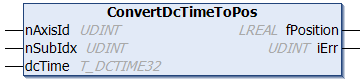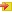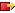# ConvertDcTimeToPosThis function block converts a 32-bit distributed clock system time variable of type T_DCTIME32 to a corresponding NC axis position (i.e. the NC axis position at precisely this time).

##Inputs

`VAR_INPUT    nAxisId : UDINT;    nSubIdx : UDINT;    dcTime  : T_DCTIME32;(* 32 bit distributed clock time *)END_VAR`

Name

Type

Description

nAxisId

UDINT

ID of the NC axis

nSubIdx

UDINT

This 32-bit input variable contains two different items of information, and is therefore divided into two 16-bit values:

• The LowWord (the least significant 16 bits) contains the subindex for relative addressing of an encoder subelement at an axis. The subindex is counted upwards from zero. For the typical case of an axis that has just one encoder, the null subindex is correct.
• The HighWord (the most significant 16 bits) contains a control word (bit mask) that affects the way in which the position is calculated (e.g. the type of interpolation or extrapolation). A bit mask value of 0x0001 means that the set acceleration of the axis is to be included in the calculation.

dcTime

T_DCTIME32

32-bit "Distributed Clock System Time" variable. This input variable is converted into the corresponding NC axis position.The 32-bit time may only be used in the narrow range of ± 2,147 seconds around the current system time, to ensure that it is unambiguous. Within the function block this prerequisite cannot be checked.

##Outputs

`VAR_OUTPUT    fPosition : LREAL;    iErr      : UDINT;END_VAR`

Name

Type

Description

fPosition

LREAL

Supplies the NC axis position corresponding to dcTime. This is an NC axis position that has been scaled and provided with an offset, having, for instance, physical units of degrees or of millimeters.

iErr

UDINT

Returns the error number if an error occurs, e.g. error 0x4012 (axis ID is not allowed, or axis does not exist within the system).

## Requirements

Development environment

Target platform

PLC libraries to include

TwinCAT v3.1.0

PC or CX (x86, x64, ARM)

Tc2_EtherCAT